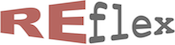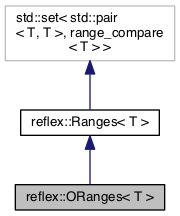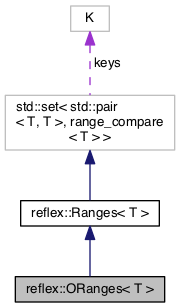reflex::ORanges< T > Class Template Reference updated Mon Jan 23 2023 by Robert van Engelen
reflex::ORanges< T > Class Template Reference

RE/flex ORanges (open-ended, ordinal value range) template class. More...

`#include <ranges.h>`

Inheritance diagram for reflex::ORanges< T >:[legend]
Collaboration diagram for reflex::ORanges< T >:[legend]

## Public Types

typedef T bound_type
Type of the bounds. More...

typedef std::set< std::pair< T, T >, range_compare< T > > container_type
Synonym type defining the base class container std::set. More...

typedef container_type::value_type value_type
Synonym type defining the base class container std::set::value_type. More...

typedef container_type::key_compare key_compare
Synonym type defining the key/value comparison std::set::key_compare. More...

typedef container_type::value_compare value_compare

typedef container_type::iterator iterator
Synonym type defining the base class container std::set::iterator. More...

typedef container_type::const_iterator const_iterator
Synonym type defining the base class container std::set::const_iterator. More...Public Types inherited from reflex::Ranges< T >
typedef T bound_type
Type of the bounds. More...

typedef std::set< std::pair< T, T >, range_compare< T > > container_type
Synonym type defining the base class container std::set. More...

typedef container_type::value_type value_type
Synonym type defining the base class container std::set::value_type. More...

typedef container_type::key_compare key_compare
Synonym type defining the key/value comparison std::set::key_compare. More...

typedef container_type::value_compare value_compare

typedef container_type::iterator iterator
Synonym type defining the base class container std::set::iterator. More...

typedef container_type::const_iterator const_iterator
Synonym type defining the base class container std::set::iterator. More...

## Public Member Functions

ORanges ()
Construct an empty range. More...

ORanges (const value_type &r)
Construct a copy of a range [lo,hi]. More...

ORanges (const bound_type &lo, const bound_type &hi)
Construct a range [lo,hi]. More...

ORanges (const bound_type &val)
Construct a singleton range [val,val]. More...

std::pair< iterator, bool > insert (const bound_type &lo, const bound_type &hi)
Update ranges to include range [lo,hi] by merging overlapping and adjacent ranges into one range. More...

std::pair< iterator, bool > insert (const bound_type &val)
Update ranges to include range [val,val] by merging overlapping and adjacent ranges into one range. More...

bool erase (const bound_type &lo, const bound_type &hi)
Update ranges by deleting the given range [lo,hi]. More...

bool erase (const bound_type &val)
Update ranges by deleting the given range [val,val]. More...

const_iterator find (const bound_type &lo, const bound_type &hi) const
Find the first range that overlaps the given range. More...

const_iterator find (const bound_type &val) const
Find the range that includes the given value. More...

ORangesoperator-= (const ORanges &rs)
Update ranges to remove ranges rs, has lower complexity than repeating erase(). More...

ORangesoperator&= (const ORanges &rs)
Update ranges to intersect the ranges of the given range set. More...

ORanges operator| (const ORanges &rs) const
Returns the union of two range sets. More...

ORanges operator+ (const ORanges &rs) const
Returns the union of two range sets. More...

ORanges operator- (const ORanges &rs) const
Returns the difference of two open-ended range sets. More...

ORanges operator& (const ORanges &rs) const
Returns the intersection of two open-ended range sets. More...

bool intersects (const ORanges &rs) const
Return true if this set of ranges intersects with ranges rs, i.e. this set has at least one range [lo',hi'] that overlaps with a range [lo,hi] in rs such that lo <= hi' and lo' <= hi. More...

bound_type hi () const
Return the highest value in the set of ranges (the set cannot be empty) More...Public Member Functions inherited from reflex::Ranges< T >
Ranges ()
Construct an empty range. More...

Ranges (const value_type &r)
Construct a copy of a range [lo,hi]. More...

Ranges (const bound_type &lo, const bound_type &hi)
Construct a range [lo,hi]. More...

Ranges (const bound_type &val)
Construct a singleton range [val,val]. More...

std::pair< iterator, bool > insert (const value_type &r)
Update ranges to include range [lo,hi] by merging overlapping ranges into one range. More...

std::pair< iterator, bool > insert (const bound_type &lo, const bound_type &hi)
Update ranges to include range [lo,hi] by merging overlapping ranges into one range. More...

std::pair< iterator, bool > insert (const bound_type &val)
Update ranges to include the range [val,val]. More...

const_iterator find (const bound_type &lo, const bound_type &hi) const
Find the first range [lo',hi'] that overlaps the given range [lo,hi], i.e. lo <= hi' and lo' <= hi. More...

const_iterator find (const bound_type &val) const
Find the range [lo',hi'] that includes the given value val, i.e. lo' <= val <= hi'. More...

Rangesoperator|= (const Ranges &rs)
Update ranges to insert the given range set, where this method has lower complexity than iterating insert() for each range in rs. More...

Rangesoperator+= (const Ranges &rs)
Update ranges to insert the ranges of the given range set, same as Ranges::operator|=(rs). More...

Rangesoperator&= (const Ranges &rs)
Update ranges to intersect the ranges with the given range set. More...

Ranges operator| (const Ranges &rs) const
Returns the union of two range sets. More...

Ranges operator+ (const Ranges &rs) const
Returns the union of two range sets, same as Ranges::operator|(rs). More...

Ranges operator& (const Ranges &rs) const
Returns the intersection of two range sets. More...

bool operator< (const Ranges &rs) const
True if this range set is lexicographically less than range set rs. More...

bool operator> (const Ranges &rs) const
True if this range set is lexicographically greater than range set rs. More...

bool operator<= (const Ranges &rs) const
True if this range set is lexicographically less or equal to range set rs. More...

bool operator>= (const Ranges &rs) const
True if this range set is lexicographically greater or equal to range set rs. More...

bool any () const
Return true if this set of ranges contains at least one range, i.e. is not empty. More...

bool intersects (const Ranges &rs) const
Return true if this set of ranges intersects with ranges rs, i.e. this set has at least one range [lo',hi'] that overlaps with a range [lo,hi] in rs such that lo <= hi' and lo' <= hi. More...

bool contains (const Ranges &rs) const
Return true if this set of ranges contains all ranges in rs, i.e. rs is a subset of this set which means that for each range [lo,hi] in rs, there is a range [lo',hi'] such that lo' <= lo and hi <= hi'. More...

bound_type lo () const
Return the lowest value in the set of ranges (the set cannot be empty) More...

bound_type hi () const
Return the highest value in the set of ranges (the set cannot be empty) More...

## Static Private Member Functions

static bound_type bump (bound_type val)
Bump value. More...

## Detailed Description

### template<typename T> class reflex::ORanges< T >

RE/flex ORanges (open-ended, ordinal value range) template class.

The ORanges class is an optimization of the ranges class for ordinal types, i.e. types with the property that values can be counted (enumerable, e.g. integers and enumerations).

The optimization merges adjacent ranges. Two ranges `[a,b]` and `[c,d]` are adjacent when `b+1=c`. It is safe to merge adjacent ranges over values of an ordinal type, because `[a,b](+)[b+1,c]=[a,c]` with `(+)` representing range merging (set union).

By storing open-ended ranges `[lo,hi+1)` in the ranges class container, adjacent ranges are merged automatically by the fact that the bounds of open-ended adjacent ranges overlap.

In addition to the methods inherited from the Range base class, open-ended ranges can be updated by deleting ranges from the set with:

• `bool erase(const bound_type& lo, const bound_type& hi)` erases a range from this set of open-ended ranges. Returns true if the set was updated.
• `bool erase(const bound_type& val)` erases a value from this set of open-ended ranges. Returns true if the set was updated.
• `ORanges& operator-=(const ORanges& rs)` erases ranges rs from this set of open-ended ranges. Returns reference to this object.
• `ORanges operator-(const ORanges& rs) const` returns difference, i.e. ranges rs erased from this set of open-ended ranges.

Open-ended ranges are more efficient than `std::set` when the values stored are adjacent (e.g. integers 2 and 3 are adjacent), since `std::set` stores values individually whereas open-ended ranges merges adjacent values into ranges. This lowers storage overhead and reduces insertion, deletion, and search time.

We can iterate over open-ended ranges. The iterator dereferences values are `[lo,hi+1)` pairs, i.e. `lo = i->first` and `hi = i->second - 1`.

Note that the largest value that can be stored in an open-ended range is the maximum representable value minus 1. For example, `reflex::ORanges<char>` holds values -128 to 126 and excludes 127. The macro WITH_ORANGES_CLAMPED can be defined to use this library such that the maximum value is clamped to prevent overflow, e.g. `reflex::ORanges<char> ch = 127` is clamped to 126. However, this still excludes 127 from the range set. This feature should not be required when the library is used with sufficiently wide container value types.

Example:

ints = 0; // insert 0
ints.insert(100, 200); // insert 100..200
ints.insert(300, 400); // insert 300..400
ints.insert(200, 300); // insert 200..300
std::cout << "Set of " << ints.size() << " open-ended ranges:" << std::endl;
for (reflex::ORanges<int>::const_iterator i = ints.begin(); i != ints.end(); ++i)
std::cout << "[" << i->first << "," << i->second << ")" << std::endl;
if (ints.find(200) != ints.end())
std::cout << "200 is in the set" << std::endl;
if (ints.find(99) == ints.end())
std::cout << "99 is not in the set" << std::endl;
if (ints.find(401) == ints.end())
std::cout << "401 is not in the set" << std::endl;
ints.erase(250, 350);
for (reflex::ORanges<int>::const_iterator i = ints.begin(); i != ints.end(); ++i)
std::cout << "[" << i->first << "," << i->second << ")" << std::endl;

Output:

Set of 2 open-ended ranges: [0,1) [100,401) 200 is in the set 99 is not in the set 401 is not in the set [0,1) [100,250) [351,401)

## Member Typedef Documentation

template<typename T>
 typedef T reflex::ORanges< T >::bound_type

Type of the bounds.

template<typename T>
 typedef container_type::const_iterator reflex::ORanges< T >::const_iterator

Synonym type defining the base class container std::set::const_iterator.

template<typename T>
 typedef std::set< std::pair,range_compare > reflex::ORanges< T >::container_type

Synonym type defining the base class container std::set.

template<typename T>
 typedef container_type::iterator reflex::ORanges< T >::iterator

Synonym type defining the base class container std::set::iterator.

template<typename T>
 typedef container_type::key_compare reflex::ORanges< T >::key_compare

Synonym type defining the key/value comparison std::set::key_compare.

template<typename T>
 typedef container_type::value_compare reflex::ORanges< T >::value_compare
template<typename T>
 typedef container_type::value_type reflex::ORanges< T >::value_type

Synonym type defining the base class container std::set::value_type.

## Constructor & Destructor Documentation

template<typename T>
 reflex::ORanges< T >::ORanges ( )
inline

Construct an empty range.

template<typename T>
 reflex::ORanges< T >::ORanges ( const value_type & r )
inline

Construct a copy of a range [lo,hi].

Parameters
 r range
template<typename T>
 reflex::ORanges< T >::ORanges ( const bound_type & lo, const bound_type & hi )
inline

Construct a range [lo,hi].

Parameters
 lo lower bound hi upper bound
template<typename T>
 reflex::ORanges< T >::ORanges ( const bound_type & val )
inline

Construct a singleton range [val,val].

Parameters
 val value

## Member Function Documentation

template<typename T>
 static bound_type reflex::ORanges< T >::bump ( bound_type val )
inlinestaticprivate

Bump value.

Returns
val + 1.
Parameters
 val the value to bump
template<typename T>
 bool reflex::ORanges< T >::erase ( const bound_type & lo, const bound_type & hi )
inline

Update ranges by deleting the given range [lo,hi].

Returns
true if ranges was updated.
Parameters
 lo lower bound hi upper bound
template<typename T>
 bool reflex::ORanges< T >::erase ( const bound_type & val )
inline

Update ranges by deleting the given range [val,val].

Returns
true if ranges was updated.
Parameters
 val value to delete
template<typename T>
 const_iterator reflex::ORanges< T >::find ( const bound_type & lo, const bound_type & hi ) const
inline

Find the first range that overlaps the given range.

Returns
iterator to the first range that overlaps the given range, or the end iterator.
Parameters
 lo lower bound hi upper bound
template<typename T>
 const_iterator reflex::ORanges< T >::find ( const bound_type & val ) const
inline

Find the range that includes the given value.

Returns
iterator to the range that includes the value, or the end iterator.
Parameters
 val value to search for
template<typename T>
 bound_type reflex::ORanges< T >::hi ( ) const
inline

Return the highest value in the set of ranges (the set cannot be empty)

Returns
highest value
template<typename T>
 std::pair reflex::ORanges< T >::insert ( const bound_type & lo, const bound_type & hi )
inline

Update ranges to include range [lo,hi] by merging overlapping and adjacent ranges into one range.

Returns
a pair of an iterator to the range and a flag indicating whether the range was inserted as new.
Parameters
 lo lower bound hi upper bound
template<typename T>
 std::pair reflex::ORanges< T >::insert ( const bound_type & val )
inline

Update ranges to include range [val,val] by merging overlapping and adjacent ranges into one range.

Returns
a pair of an iterator to the range and a flag indicating whether the range was inserted as new.
Parameters
 val value to insert
template<typename T>
 bool reflex::ORanges< T >::intersects ( const ORanges< T > & rs ) const
inline

Return true if this set of ranges intersects with ranges rs, i.e. this set has at least one range [lo',hi'] that overlaps with a range [lo,hi] in rs such that lo <= hi' and lo' <= hi.

Returns
true if this set intersects rs.
Parameters
 rs ranges
template<typename T>
 ORanges reflex::ORanges< T >::operator& ( const ORanges< T > & rs ) const
inline

Returns the intersection of two open-ended range sets.

Returns
the intersection of this set and rs.
Parameters
 rs ranges to intersect
template<typename T>
 ORanges& reflex::ORanges< T >::operator&= ( const ORanges< T > & rs )
inline

Update ranges to intersect the ranges of the given range set.

Returns
reference to this object.
Parameters
 rs ranges to intersect
template<typename T>
 ORanges reflex::ORanges< T >::operator+ ( const ORanges< T > & rs ) const
inline

Returns the union of two range sets.

Returns
the union of this set and rs.
Parameters
 rs ranges to merge
template<typename T>
 ORanges reflex::ORanges< T >::operator- ( const ORanges< T > & rs ) const
inline

Returns the difference of two open-ended range sets.

Returns
the difference of this set and rs.
Parameters
 rs ranges
template<typename T>
 ORanges& reflex::ORanges< T >::operator-= ( const ORanges< T > & rs )
inline

Update ranges to remove ranges rs, has lower complexity than repeating erase().

Returns
reference to this object.
template<typename T>
 ORanges reflex::ORanges< T >::operator| ( const ORanges< T > & rs ) const
inline

Returns the union of two range sets.

Returns
the union of this set and rs.
Parameters
 rs ranges to merge

The documentation for this class was generated from the following file: